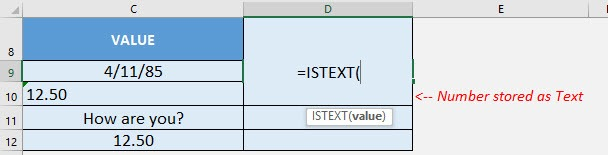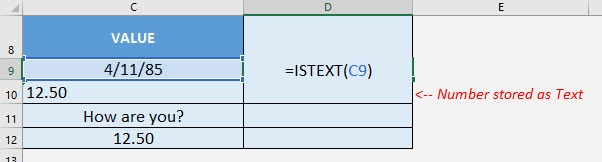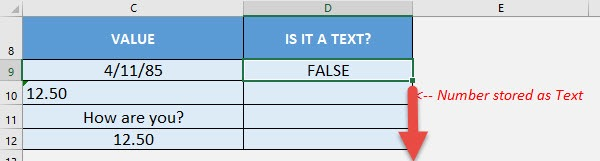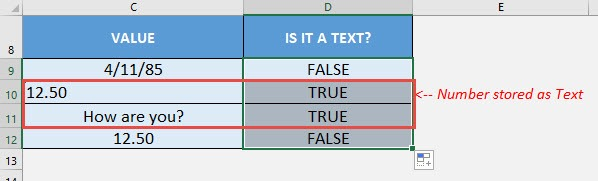What does it do?

Checks if the cell value is text or not

Formula breakdown:

=ISTEXT(value)

What it means:

=ISTEXT(value to be checked if it is a text or not)

Have a bunch of values and you need to check which ones are text? The ISTEXT Formula in Excel is perfect for this!

There are a couple of interesting points to take note of the ISTEXT Formula:

• Dates are also treated as numerical values, so the ISTEXT Formula will return FALSE
• If a number is stored as text, then the ISTEXT Formula will return TRUE

I explain how you can do this below:STEP 1: We need to enter the ISTEXT function in a blank cell:

## =ISTEXT(STEP 2: The ISTEXT arguments:

## value

What is the value to be checked?

Select the cell containing the value you want to be checked if it is a text:

## =ISTEXT(C9)Apply the same formula to the rest of the cells by dragging the lower right corner downwards.You can now see which ones are text!How to Use the ISTEXT Formula in Excel